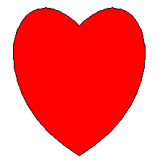deutsch     english    français     Print

## 3.8 STRING ART### INTRODUCTION

 You have likely played with string art already in preschool. For this, you hammered nails or inserted needles into a timber or carton board to create a particular figure, according to a crafting guide. Most of these were arranged at equal intervals and you linked them together with threads. When you placed a sufficient amount of threads, interesting curves appeared where the thread consolidated. In mathematics, this is called envelope (also envelope curve) because the threads are tangent to this curve.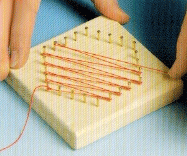From Täubner, Walz: Fadengrafik

Instead of creating the thread graphic yourself, you can also instruct a machine to do it. This would require the machine to not only understand the instructions but then to also translate these instructions into an action, for example using a robot arm to pull the strings or record the strings on a screen. Such an instruction manual for a machine is also called an algorithm. You can first formulate the algorithm as a “craft” instruction understandable in colloquial language. Since it is desirable that the machine produces the exact same pattern on each pass, the algorithm must be formulated so precisely that the machine knows exactly what to do at every step. Programming languages were invented for this and that is why you learn to program, since in the natural languages there is no such unambiguity.

PROGRAMMING CONCEPTS: Algorithm, data structure, model, program elegance, list, index

### POINTS AS LISTS

 Instead of working with boards, nails, and threads, you can transfer the procedure to your computer. Thereby you make an portrayal of nature, you model the board as a screen window, the nails as points on the screen, and the threads as lines. In transferring the algorithm into a programming language, it is important to establish the closest relationship possible to reality. Nails, and geometric points respectively, represent tangible objects to you, and so they should be in the program as well.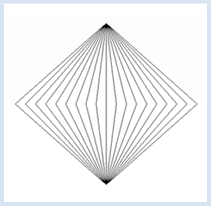In geometry, you can write P(x, y) for a point, where x and y are the coordinates. In the program, we can pack the two numbers x and y into a data structure, called a list. We write  p = [x, y]. The geometric point P(0, 8) is thus modeled by the list p = [0, 8] .

You can access the individual components of a list with an index with a count starting at 0. You have to write the index in a set of square brackets, so p for the x-coordinate, and p for the y-coordinate.

The nice thing is that all of the graphic functions of the GPanel are "list conscious" because they also work with point lists instead of x-y-coordinates. Your program models the pulling of threads from nail A around 19 nails at the coordinates on the x-axis to nail B, and back again. You can even incorporate a delay() which causes the stringing to take a longer time that is graspable by humans.

```from gpanel import *

DELAY = 100

def step(x):
p1 = [x, 0]
draw(p1)
delay(DELAY)
draw(pB)
delay(DELAY)
p2 = [x + 1, 0]
draw(p2)
delay(DELAY)
draw(pA)
delay(DELAY)

makeGPanel(-10, 10, -10, 10)
pA = [0, 8]
pB = [0, -8]
move(pA)
for x in range(-9, 9, 2):
step(x)
```
Highlight program code (Ctrl+C copy, Ctrl+V paste)

### MEMO

 The data must also be structured conveniently in the implementation of an algorithm. Our geometric points are modeled as a list with two elements (x- and y-coordinates). The choice of the data structure significantly affects the program. Niklaus Wirth, a famous computer science professor at the ETH Zürich, aptly said: program = algorithm + data structure [Ref.] Lists can store multiple values, named list elements. They are defined with square brackets. You can read the individual elements with a list index and assign new values. All of the graphics commands of GPanel also work with points modeled as lists of x- and y-coordinates.

### PROGRAMMING IS AN ART

 You probably realize that you can create the previous thread graphic much easier if you draw the lines independently of how the thread would actually be drawn by hand. You just need to connect the points A and B with routes. ```from gpanel import * makeGPanel(-10, 10, -10, 10) pA = [0, 8] pB = [0, -8] for x in range(-9, 10, 1): pX = [x, 0] line(pA, pX) line(pB, pX) ```Highlight program code (Ctrl+C copy, Ctrl+V paste)

### MEMO

 An algorithm can be implemented in various ways that differ in length of code and duration of the execution of the program. We also speak of more elegant and less elegant programs. Just remember that it is not enough for a program to produce a correct result, but it should also be written elegantly. Consider programming an art!

###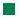You often need dividing points of a line segment for string art. For this there is a simple function in GPanel called getDividingPoint(pA, pB, r), which you pass the two endpoints pA and pB of the line and the division factor r. It returns you the dividing point as a list. You are now modeling a thread graphic with nails on the sides AB and AC with an especially elegant program.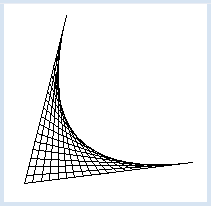```from gpanel import *

makeGPanel(0, 100, 0, 100)

pA = [10, 10]
pB = [90, 20]
pC = [30, 90]

line(pA, pB)
line(pA, pC)

r = 0
while r <= 1:
pX1 = getDividingPoint(pA, pB, r)
pX2 = getDividingPoint(pA, pC, 1 - r)
line(pX1, pX2)
r += 0.05
delay(300)
```
Highlight program code (Ctrl+C copy, Ctrl+V paste)

### MEMO

 Library functions such as getDividingPoint() can greatly simplify a program. For certain well-defined tasks, you should use existing library functions that you know from your programming experience here, taken from documentations, or from what you can find on the Web. Mathematically, the resulting curve is a quadratic Bézier curve. You can draw it with the function quadraticBezier(pB, pA, pC), where pB and pC are the endpoints, and pA is the control point of the curve.

###Modeling natural processes with the computer is not just a game, it also has versatile applications. You can test different situations in a much shorter time and with much less effort with a computer until you have found one that you want to implement into practice. Your program is particularly attractive if you can make changes with the mouse that have an immediate effect. With Python, this can be incorporated with little extra effort, by using callbacks. In your program, you can move the vertex A by moving the mouse, and a new thread graphic will be made immediately.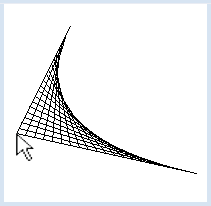In order to create the graphics, you use the function updateGraphics() which is called by the mouse callbacks. Every time you delete the entire graphics window and then recreate it with point A at the current location of the mouse cursor.

```from gpanel import *

def updateGraphics():
clear()
line(pA, pB)
line(pA, pC)
r = 0
while r <= 1:
pX1 = getDividingPoint(pA, pB, r)
pX2 = getDividingPoint(pA, pC, 1 - r)
line(pX1, pX2)
r += 0.05

def myCallback(x, y):
pA = x
pA = y
updateGraphics()

makeGPanel(0, 100, 0, 100,
mousePressed = myCallback,
mouseDragged = myCallback)

pA = [10, 10]
pB = [90, 20]
pC = [30, 90]
updateGraphics()
```
Highlight program code (Ctrl+C copy, Ctrl+V paste)

### MEMO

 You can also deal with two different events, here the press event and the drag event, using the same callback.

### EXERCISES

1.

 Create the adjacent thread graphic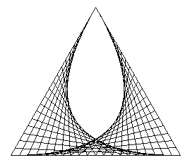2.

 Work from the thread graphic from exercise 1, so that you can draw the top of the triangle with a mouse drag and the graphic is repeatedly drawn anew.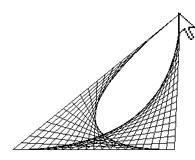### BÉZIER CURVES

These curves were invented in the sixties of the last century by Pierre Bézier, then an engineer of the car company Renault, so one could produce aesthetically pleasing curves for the design of industrial products.

You can create a cubic Bézier curve as a thread graphic using the De Casteljau algorithm.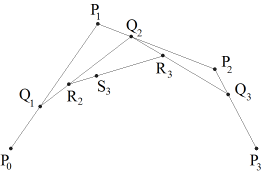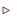Specify 4 points P0, P1, P2, P3. (P0 and P3 will be the end points of the curve, P1 and P2 so-called control points)Connect P0P1, P1P2, P2P3The routes P0P1, P1P2, P2P3 are arranged into equidistant division points. For a given division ratio, this results in the dividing points Q1, Q2, Q3Connect Q1Q2, Q2Q3Split the routes Q1Q2, Q2Q3 in the same division/factor ratio. This results in the division points R2 and R3Connect R2R3

You can easily implement the algorithm into a program if you implement the points as lists and call the function getDividingPoint() several times.

```from gpanel import *

makeGPanel(0, 100, 0, 100)

pt1 = [10, 10]
pc1 = [20, 90]
pc2 = [70, 70]
pt2 = [90, 20]

setColor("green")

line(pt1, pc1)
line(pt2, pc2)
line(pc1, pc2)

r = 0
while r <= 1:
q1 = getDividingPoint(pt1, pc1, r)
q2 = getDividingPoint(pc1, pc2, r)
q3 = getDividingPoint(pc2, pt2, r)
line(q1, q2)
line(q2, q3)
r2 = getDividingPoint(q1, q2, r)
r3 = getDividingPoint(q2, q3, r)
line(r2, r3)
r += 0.05

setColor("black")
#cubicBezier(pt1, pc1, pc2, pt2)
```
Highlight program code (Ctrl+C copy, Ctrl+V paste)

### MEMO

 A cubic Bézier curve is defined by 4 points. You can draw one in GPanel with the function cubicBezier(). The current drawing color and line thickness will be used.

### INTERACTIVE CURVE DESIGN

 Combining your knowledge, you can already write a quite professional program with which you can create a Bézier curve and interactively change it with the mouse. The program even notices when you are near one of the 4 points with the cursor and colors it. With a press event you can then grab the point and move it around. The four points must be run through multiple times in the program. It is therefore advisable that you also put them in a list with the name points, so that you can process them with a for structure.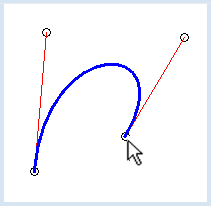It is also important that you know which of the points you have just grabbed. You store this information in the variable active: if none of the points are grabbed it has the value -1, otherwise its value corresponds to the index of the corresponding point.

```from gpanel import *

def updateGraphics():
# erase all
clear()

# draw points
lineWidth(1)
for i in range(4):
move(points[i])
if active == i:
setColor("green")
fillCircle(2)
setColor("black")
circle(2)

# draw tangents
setColor("red")
line(points, points)
line(points, points)

# draw Bezier curve
setColor("blue")
lineWidth(3)
cubicBezier(points, points, points, points)

def onMouseDragged(x, y):
if active == -1:
return
points[active] = x
points[active] = y
updateGraphics()

def onMouseReleased(x, y):
active = -1
updateGraphics()

def onMouseMoved(x, y):
global active
active = near(x, y)
updateGraphics()

def near(x, y):
for i in range(4):
rsquare = (x - points[i]) * (x - points[i]) +
(y - points[i]) * (y - points[i])
if rsquare < 4:
return i
return -1

pt1 = [20, 20]
pc1 = [10, 80]
pc2 = [90, 80]
pt2 = [80, 20]
points = [pt1, pc1, pc2, pt2]
active = -1

makeGPanel(0, 100, 0, 100,
mouseDragged = onMouseDragged,
mouseReleased = onMouseReleased,
mouseMoved = onMouseMoved)
updateGraphics()
```
Highlight program code (Ctrl+C copy, Ctrl+V paste)

### MEMO

 There are also complicated data structures such as lists whose elements are again lists. For example, you can address the x-coordinate of P1 using the points, thus with double brackets. Today, Bézier curves are important design tools in the CAD domain [Ref.]

### EXERCISES

1.

 The heart consists of two cubic Bézier curves with the same start and end points, plus symmetrical control points. On a piece of paper, draw a sketch of where these points should be placed and then create the drawing. The filling is made with the function fill(point, old_color, new_color), where point stands for an inner point of a bordered area.